#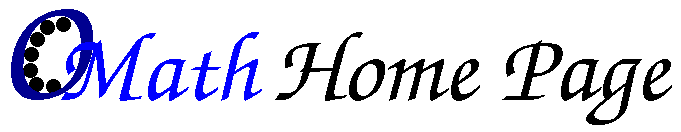Please check also the OpenModelica Modeling and Simulation Environment.

ObjectMath is a programming and modeling environment for object oriented mathematical modeling and generating efficient C++ or Fortran90 code for use in simulation applications, mostly (numerical, scientific) computing.
This system partly automates the conventional approach of hand translation from mathematical models to numerical simulation code e.g. in Fortran. The ObjectMath language is an object oriented extension to the Mathematica computer algebra language, which provides mathematical notation and symbolic transformations. Thus, the ObjectMath programming environment offers the following:
ObjectMath is designed and supported by a team at PELAB (Programming Environment Lab), Linköping University, Sweden. Some of the new developments of ObjectMath are part of the Modelica modeling language design effort.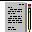There is a collection of papers on various aspects of ObjectMath.

#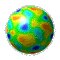ObjectMath - the Process of Mathematical Modeling and Software Development

#### How we go from a initial model to the development of simulation applications ?

• The user has physical application oriented knowledge
• Express this as a mathematical, object-oriented specification
• This specification is expressed in ObjectMath
• Possibly perform symbolic transformations of formulas and equations
• Execute it and obtain results (which may cause adjustments of the specification)
• Graphically present the results (which gives understanding of the problem that often causes changes to the specifification)

#### How is software development of a simulation application supported?

• We specify the simulation problem through mathematical modeling.
• Possibly execute interpretively within Mathematica (for small problems)
• Perform model transformations (using the Mathematica computer algebra system)
• Automatically generate code (C++, Fortran90, parallel code - HPF)
• Specify input data for numerical experiments
• Run the experiment
• Visualize the data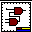See roadmap of software development with ObjectMath.

#ObjectMath - An Object-oriented Computer Algebra Language

ObjectMath is an Object-Oriented extension to Mathematica, a computer algebra language from Wolfram Research . Mathematica functions and equations can be grouped into classes, in order to structure the mathematical model. Equations and functions can be inherited from general classes into more specific classes, which allows reuse within the mathematical model. Below are two example models.
You can see classes and instances in these examples, as well as inheritance relations between them (including single and multiple inheritance). The part-of relation allows structured objects to be expressed as a composition of parts. The ObjectMath class browser can show both inheritance relations and part-of relations.

Inheritance allows formulae and equations to be reused. (For large models the amount of ObjectMath code is reduced approximately three times through reuse as compared to expressing the model in standard Mathematica).

This object oriented way of modeling is a natural way to describe physical systems.

#ObjectMath - a programming environment

The programming environment include the following components:
• The ObjectMath language - including object-oriented features and type declarations. The language is integrated with Mathematica.
• The code is edited by
• class browser/editor
• text editor
• Code generator to produce Optimized C++ and Fortran90 code
• Form based data entry tool to specify numerical experiments
• Visualization tool to draw curves and perform 3D graphics animations based on simulation resultsSee how the internal components of the environment are connected.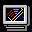See small screendump of the environmentSee big screendump of the environment

#ObjectMath - generating efficient code

• The equations, formulae and functions are symbolically transformed at compile or design time. Part of the computation is eliminated through symbolic transformations, which yield partially reduced symbolic expressions.The rest is computed numerically at run-time. Remaining ordinary differential equations are solved numerically at run-time.
• Code for numerical computation is generated
• Optimizations:
• common subexpression elimination
• constant folding
• unfolding for some functions
• The code is linked together with library routines, e.g. for i/O and numeric ODE solution.

### Efficiency:

A two-body example:
• Pure Mathematica code - 6 minutes
• Generated C++ code - 10 sec
• Generated C++ code with common subexpression elimination - 1 sec

### Applications to realistic models:

A mathematical model of surface interaction have been designed in cooperation with SKF.
• 50% of implementation is generated from ObjectMath.
• 147 KB ObjectMath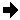581 KB Mathematica546 KB C++ code

### Parallel code generator

This part of the system is more experimental, and has not been used in industrial applications. There are two approaches to Extracting Parallelism from Mathematical Models (so far in ObjectMath primarily for ODEs):
• Parallelism at the equation system level
• Analyze dependencies between equations
• Find partly independent Strongly Connected Components (SCCs)
• The SCCs form subsystems of equations that can be solved partly in parallel or in pipeline
•see the scheme of partitioning and example
• Parallelism at the equation levelSee also papers [1,2,10,11,12,13]

#Scientific visualisation using ObjectMath

We generate efficient C++/Fortran90 code from ObjectMath models which include geometry descriptions expressed as parametric surfaces. This code is linked together with a powerful 3D browsing environment which uses OpenGL with possible hardware support, e.g. Creator 24bit 3D graphics on UltraSparc workstations.See screendump of the BEAST environment (GIF,color,85K) (PS, color 100K) (PS, B/W 100K)See also papers 

# The ObjectMath team

ObjectMath has been designed at the PELAB laboratory at Dept. of Computer and Information Science , Linköping University, Linköping,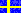Sweden . The team has the following members:
• Peter Fritzson, petfr@ida.liu.se(Project leader and the main contact person. Design of ObjectMath 5/Modelica. Programming environment.)
• Vadim Engelson (Programming environment and visualization/3Danimation. Code generation.)
• Johan Gunnarsson (Dept. of Electrical Engineering, ISY) (Design of ObjectMath 5)
• Patrik Nordling (Bearing simulation applications using parallel computing)
• Tommy Persson (Applications in scientific computing. System support)
• Patrik Hägglund ( Model design, comparative analysis of computer algebra languages)
Ex-members:
• Niclas Andersson (Parallel code generation, parallel computing platforms)
• Rickard Westman (ObjectMath language and environment, networking)
• Lars Viklund (Programming environment, networking, parallel computing platforms)
• Lars Willför (Sequential code generation)
Questions about availability of the implementation should be directed to Peter Fritzson.

Visit collection of ObjectMath papers and other documents